# Theorems of Inequality

Lesson Transcript
Instructor: Usha Bhakuni

Usha has taught high school level Math and has master's degree in Finance

In trigonometry, inequality theorems describe the relationships between the sides and angles of a triangle. Discover the theorems of inequality, including the theorems of exterior angle inequality, triangle inequality, and angle-side relationship. Updated: 12/14/2021

## Triangles & Inequalities

Triangles are one of the most common geometrical shapes found around us. They consist of three sides containing three angles within. So let's explore some geometrical theorems related to inequalities in triangles.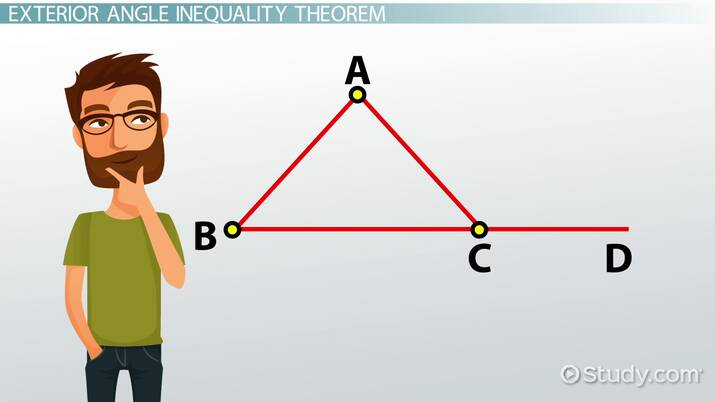An error occurred trying to load this video.

Try refreshing the page, or contact customer support.

Coming up next: Triangle Inequality: Theorem & Proofs

### You're on a roll. Keep up the good work!

Replay
Your next lesson will play in 10 seconds
• 0:04 Triangles & Inequalities
• 0:18 Exterior Angle…
• 1:07 Triangle Inequality Theorem
• 2:19 Angle-Side Relationship
• 3:37 Lesson Summary
Save Save

Want to watch this again later?

Timeline
Autoplay
Autoplay
Speed Speed

## Exterior Angle Inequality Theorem

An exterior angle of a triangle is the angle formed between any side of the triangle and an external extension of the adjacent side. There are six external angles formed in a triangle, two at each of the vertices.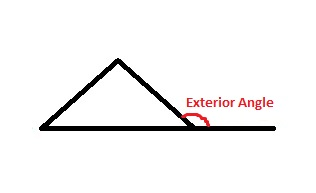The exterior angle inequality theorem states that the measure of any exterior angle of a triangle is greater than both of the non-adjacent interior angles. This rule is satisfied by all the six external angles of a triangle.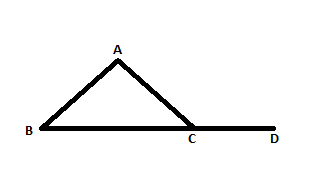In the image appearing on your screen, we can see that ∠ACD is an external angle. So the measure of ∠ACD > ∠CAB and the measure of ∠ACD > ∠CBA.

## Triangle Inequality Theorem

A triangle can't be formed by just any set of three random lines. All triangles must observe the triangle inequality theorem. It states that the sum of lengths of two sides of the triangle will always be greater than the length of the third side. This rule is satisfied by all the three sides of a triangle. It means if we have the lengths of two sides, we can safely bet that the length of the third side will be smaller than the sum of the two sides.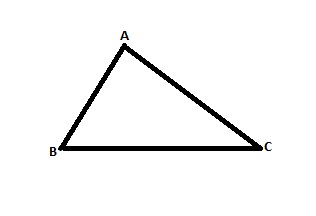In the image on your screen, there are three inequalities:

1. AB + BC > AC
2. AB + AC > BC
3. BC + AC > AB

Let's try to draw an imaginary triangle with sides of 3 cm, 4 cm, and 10 cm. We can see that 4 + 10 > 3, 3 + 10 > 4, but 4 + 3 < 10. Therefore, the sides with these dimensions don't follow the triangle inequality theorem. This is why we can confidently say that a triangle like this can't exist.

## Angle-Side Relationship

In a triangle, the largest side in a triangle will be opposite to the largest angle and the shortest side will be opposite to the smallest angle, known as the angle-side relationship. Let's look at the angle-side relationship in a triangle that has three unequal sides, with AC being the smallest and BC being the longest.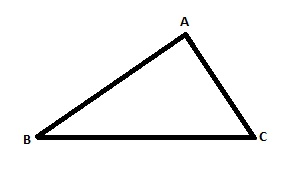To unlock this lesson you must be a Study.com Member.

### Register to view this lesson

Are you a student or a teacher?